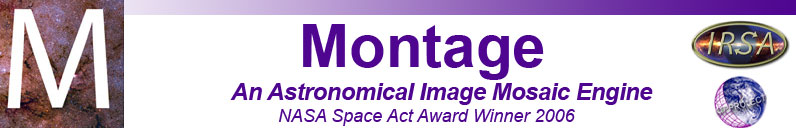# mFixNan

Description:
Converts NaNs found in the image to some other value (given by the user), or converts a range of supplied values into NaNs.
Syntax:
mFixNaN [-d level] [-v NaN-value] in.fits out.fits [minblank maxblank]

Switches:

-d level
Turn on debugging to the specified level (1-3)
-b
Check for non-physical boundary area (e.g. the corners of an Aitoff image) and correct them.
-v NaN-value
Value to use in place of any NaNs

Arguments:

in.fits
Input FITS image file
out.fits
Path of output FITS file. To run in "count" mode without creating an output file, use a dash ("-") for this argument.
minblank maxblank
If the "-v" switch is not used, mFixNaN will replace all pixel values between minblank and maxblank with NaN.
Results:
[struct stat="OK", rangeCount=rangeCount, nanCount=nanCount]
rangeCount is the number of pixels that were found between minblank and maxblank, if they were specified. If not (i.e., NaNs were removed and replaced with value), nanCount is the number of NaNs removed.

Examples:

A FITS image with BITPIX -64 (double-precision floating point) was generated without using NaNs; all "blank" pixels are represented by very small negative numbers. This can throw off initial attempts to display the image with a proper stretch, and does not conform to the FITS standard. To replace all those "blank" pixels with NaNs:

mFixNaN original.fits NaN.fits -4.61169e32 -4.61169e10
[struct stat="OK", rangeCount=1321, nanCount=0]

To convert those NaNs back into a single pixel value:

mFixNaN -v -4.6e32 NaN.fits blankval.fits
[struct stat="OK", rangeCount=0, nanCount=1321]

Return Codes:

• [struct stat="OK", rangeCount=rangeCount, nanCount=nanCount"]
• [struct stat="ERROR", msg="Usage: mFixNaN [-d level] [-v NaN-value] in.fits out.fits [minblank maxblank] (output file name '-' means no file)"]
• [struct stat="ERROR", msg="No debug level given"]
• [struct stat="ERROR", msg="Debug level string is invalid: level"]
• [struct stat="ERROR", msg="Debug level string is invalid: level"]
• [struct stat="ERROR", msg="Debug level string cannot be negative"]
• [struct stat="ERROR", msg="No value given for NaN conversion"]
• [struct stat="ERROR", msg="NaN conversion value string is invalid: 'NaN-value'"]
• [struct stat="ERROR", msg="Invalid input file 'in.fits']
• [struct stat="ERROR", msg="min blank value string is not a number"]
• [struct stat="ERROR", msg="max blank value string is not a number"]
• [struct stat="ERROR", msg="Image file in.fits missing or invalid FITS"]
• [struct stat="ERROR", status=errnum, msg="FITS library error"]